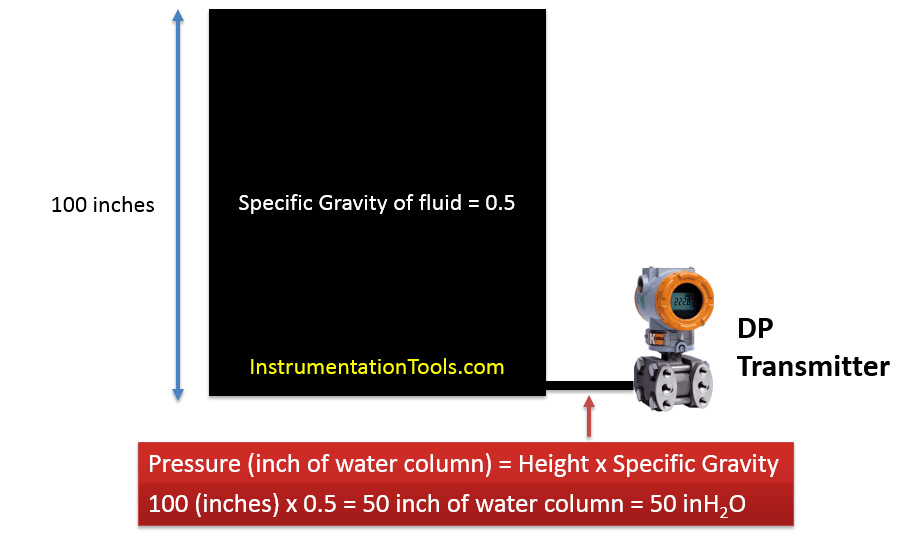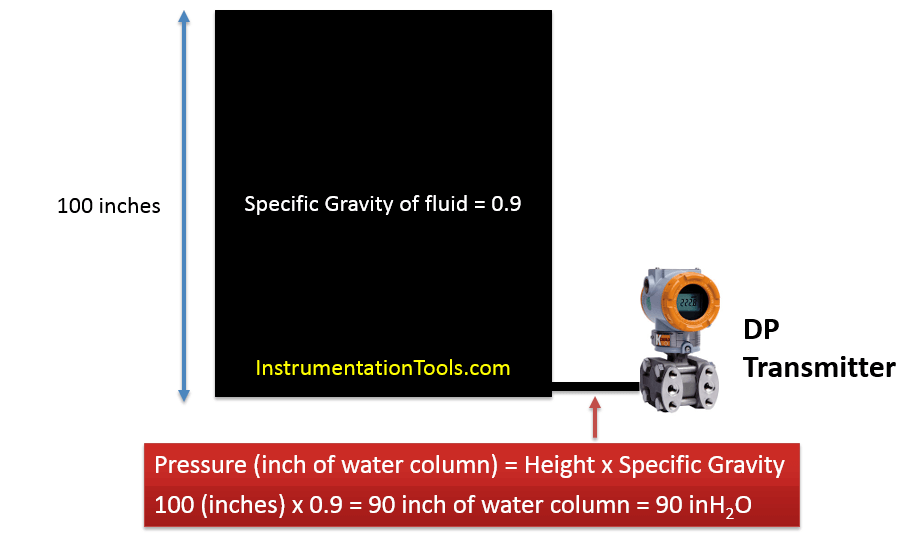# How to Measure Density using DP Transmitter?

For Density measurement using DP transmitter, the height of the tank is kept constant then Change in Pressure is due to Change in Density.

First Let’s Understand the concept

The calculation is very similar to Normal level calculation

## Density Calculation​ Using DP Transmitter

Here’s our simple pressure measurement formula.The Pic above shows a Container of Height 100 Inches

And this tank contains Liquid of Specific gravity 0.5

So what is the pressure at the bottom?

Height x Specific gravity= InH2O ……..(Reference below)

100 (inches) X 0.5 = 50 inH2O

Now let’s keep the “Height Same” and Change specific Gravity to 0.9

Height x Specific gravity= InH2O

100 (inches) X 0.9 = 90 inH2OSo if Height is kept CONSTANT then change in pressure is just due to CHANGE IN DENSITY (Specific gravity)

When the Transmitter feels the pressure of 50inH2O we get to know the S.G of liquid inside is 0.5

And, When Transmitter feels the pressure of 90inH2O we get to know the S.G of liquid inside is 0.9

If this Concept is clear the calculation is “Piece of Cake” !!!

### Our Calibration range is 50 inH2O to 90 inH2O

Here are the steps in a simplified manner

#### For 4mA Cases (LRV – Lower Range value case)

1) Calculate the Pressure at LP side (tapping) of Transmitter

2) Calculate the Pressure at HP side (tapping) of Transmitter

3)Differential pressure = Pressure at (HP Side – LP Side)

#### Repeat the same for 20mA case (URV – Upper Range value case)

Reference:-

Refer Simple Level calculation article for reference as to how to execute the above steps

Thanks for Reading !!